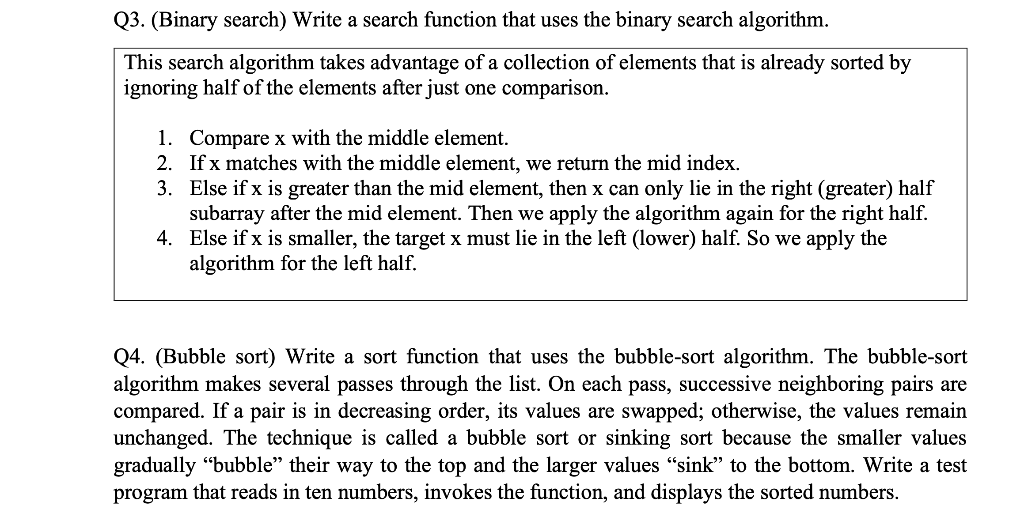# (Solved): how to solve this question with python This search algorithm takes advantage of a collection of ele ...how to solve this question with python

This search algorithm takes advantage of a collection of elements that is already sorted by ignoring half of the elements after just one comparison. 1. Compare $$\mathrm{x}$$ with the middle element. 2. If $$\mathrm{x}$$ matches with the middle element, we return the mid index. 3. Else if $$\mathrm{x}$$ is greater than the mid element, then $$\mathrm{x}$$ can only lie in the right (greater) half subarray after the mid element. Then we apply the algorithm again for the right half. 4. Else if $$x$$ is smaller, the target $$x$$ must lie in the left (lower) half. So we apply the algorithm for the left half. Q4. (Bubble sort) Write a sort function that uses the bubble-sort algorithm. The bubble-sort algorithm makes several passes through the list. On each pass, successive neighboring pairs are compared. If a pair is in decreasing order, its values are swapped; otherwise, the values remain unchanged. The technique is called a bubble sort or sinking sort because the smaller values gradually "bubble" their way to the top and the larger values "sink" to the bottom. Write a test program that reads in ten numbers, invokes the function, and displays the sorted numbers.

We have an Answer from Expert